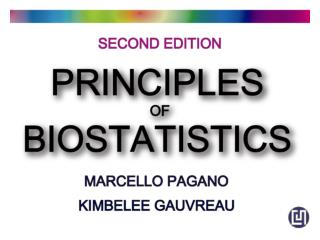DownloadDownload PresentationAnalysis of Variance

# Analysis of Variance

Télécharger la présentation## Analysis of Variance

- - - - - - - - - - - - - - - - - - - - - - - - - - - E N D - - - - - - - - - - - - - - - - - - - - - - - - - - -
##### Presentation Transcript

1. 12 Analysis of Variance

2. Analysis of variance (ANOVA) • How do we compare multiple samples at once? • We could do pairwise t-tests to see which differed from one another. • However, the a-level probability of making an error applies to each test. So, the real chance of making anerror is increasedby using multiple tests. • There are ways of dealing with this, but it is time-consuming to do many pairs. • The analysis of variance procedure (called ANOVA) is a way to make multiple comparisons. • H0 : m1 = m2 = m3 = .... mn, for n means • Ha : at least one mean is not equal to the others, i.e. mi ≠ mj , ormj ≠ mk or mi ≠ mj ≠ mk or more http://www.tnstate.edu/ganter/BIO%20311%20Ch%2011%20ManyMeans.html

3. Group i j obs. x11 x12 . . . n1 n2 n3 . . . . x1.x2. Total number of obs. Group average Overall mean, n* x.. Analysis of variance (ANOVA) Some necessary definitions and notation • xij= observation j in group i • I = the number of groups • ni= the sample size of group i • Dot notation = a dot that replaces an index stands for the mean for the observations the dot replaces. • xi• = mean for group i (the j's have been averaged for the group) • In summation notation, the dot looks like: • The total number of observations is n* : • the OVERALL MEAN x••

4. Analysis of variance (ANOVA) • Now we need to define some of the terms that will be important for this technique. • The first term is called a "SUM OF SQUARES" (abbreviated "SS"), SUM OF SQUARES: TOTAL, WITHIN GROUPS, AND BETWEEN GROUPS • SS(total) means the sum of squares for all of the data, corrected for the OVERALL MEAN OF ALL OBSERVATIONS. • SS(within groups) means the sum of squares for all of the groups, corrected for the MEAN OF EACH GROUP(i.e. j observations) • The SS(between groups) is • the degrees of freedom (d.o.f) associated with the total n* -1 • the degrees of freedom associated with the within groups is n* - I • the degrees of freedom associated with the between groups is I -1.

5. Analysis of variance (ANOVA) • the relationship between the Sum of Squares we have just calculated: • SS(total) = SS(between groups) + SS(within groups) • Another way to write this (in terms of an experiment) is: • SS(total) = SS(treatments) + SS(random error) • The mean squares • If SS(total) is fixed (= can't change), then as SS(treatments) increases, SS(randomerror) must decrease. • A successful experimenthas most of the sums of squares in the between group partition, so that groups differ(treatments differ from control, etc.). • An unsuccessful experiment has most of the sums of squares in the within group partition, so that it doesn't matter which group an observation is in (treatment means are the same as control, etc.).

6. Analysis of variance (ANOVA) A summary of presenting the calculated values.

7. Analysis of variance (ANOVA) Example – do the three groups have the same mean values ? H0 : m1 = m2 = m3 reject null hypothesis, since p<0.05 3.89 < 4.43 EXCEL functions – SUMXMY2, FINV, AVERAGE

8. Analysis of variance (ANOVA) – using Excel tool reject null hypothesis, since p<0.05

9. Chapter12 p288

10. Chapter12 p290

11. Chapter12 p291